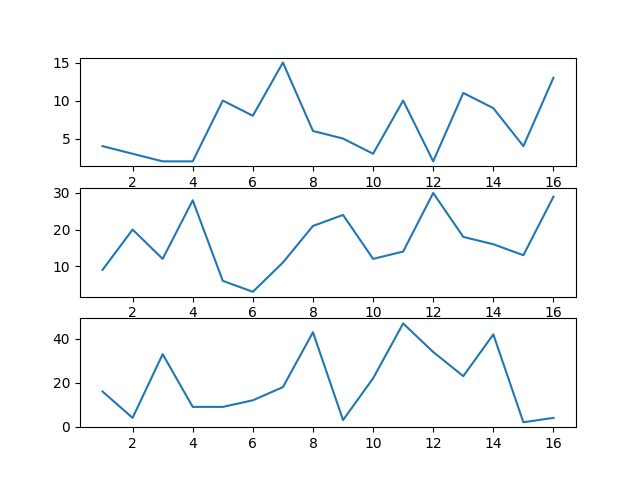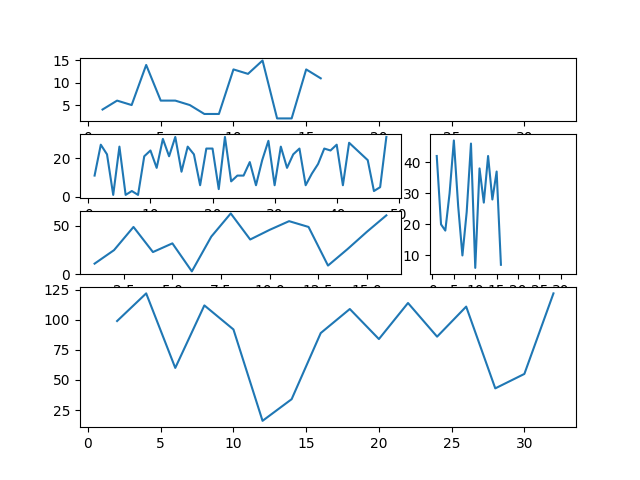# How to use subplots in matplotlib DataVisualization using python – part 2

I have already written about using subplots using matplot lib in this article. While working further on matplotlib, I found more convenient and easy to understand way for doing the same thing hence I decide to put part of of this port.

Here we are going to use subplot2grid() . It is a helper function that is similar to subplot() but uses 0-based indexing and let subplot to occupy multiple cells. The grid is specified by shape, at location of loc, spanning rowspan, colspan cells in each direction.

subplot2grid(shape, loc, rowspan=1, colspan=1,sharex=ax1)

Let us get our hands dirty. Below is simple code to plot three charts on the same plot.

Here is the outputHere a bit complex subplots

Here is the outputCode for generating plot is as below

ax5 = plt.subplot2grid((5,3), (3, 0), colspan=3,rowspan=2,sharex=ax1)
• First bracket (5,3) indicates the number of rows and columns on the graph
• second bracket (3,0) indicates location by row, column. Since this is zero based indexing, (0,0) indicates first row and first column
• Plot can cover multiple rows and columns and can be specided by using colspan and rowspan
• Axis can be shared between two plots and its indicated by sharex

This site uses Akismet to reduce spam. Learn how your comment data is processed.